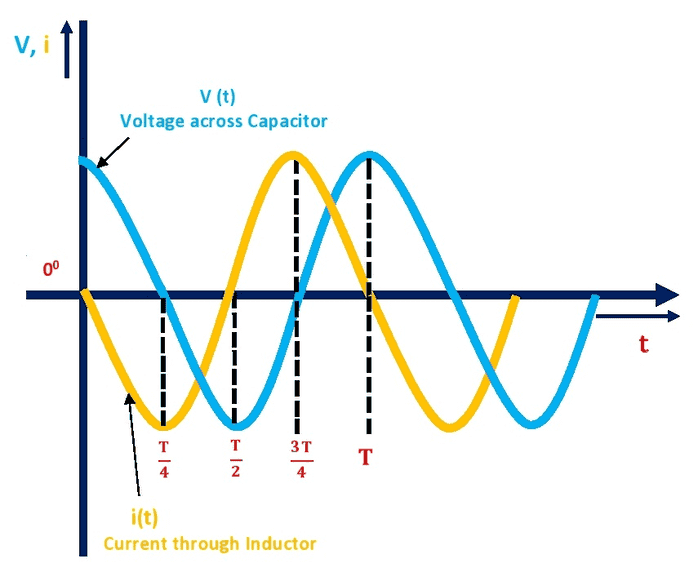# LC circuit current

• I
lukka98
Without mathematical formulas, but only with a "Physical intuitive meaning", why if at t=0, I have a charged capacitor, and I connect it through a wire ,forming a closed path, to a inductor the current increasing with time and his derivative decreasing?

To me seems like the inductor oppose "to the flow" at maximum initially, and then it allow the current to flow greater and greater but with slow increasing until its maximum, then the current cannot drop down instantly to zero so an emf rising and with decreasing current charge the capacitor but opposite in sign, and then the cycle continue.
I can say is just a conservation of energy between magnetic(L) and potential(C), but I cannot understand why the current has the behavior it has.

I said with no formulas because I have understand how to find all about LC with math, but I don't understand how happen physically.

thanks.

•Delta2

Mentor
I said with no formulas because I have understand how to find all about LC with math, but I don't understand how happen physically.
Can you show us the two differential equations relating voltage and current for inductors and capacitors? If you really do understand those two equations and how they govern the trading back-and-forth of the energy storage in a lossless parallel LC "tank" circuit, then you should have a good intuition for the physical situation.

It's similar to the Simple Harmonic Motion (SHM) of a mass hanging on a spring. When you stretch the mass/spring out a bit and let it go, what happens?•DaveE and vanhees71
lukka98
Can you show us the two differential equations relating voltage and current for inductors and capacitors? If you really do understand those two equations and how they govern the trading back-and-forth of the energy storage in a lossless parallel LC "tank" circuit, then you should have a good intuition for the physical situation.

It's similar to the Simple Harmonic Motion (SHM) of a mass hanging on a spring. When you stretch the mass/spring out a bit and let it go, what happens?I solve it using Laplace transformation, and "analyzing" the T(s), so at the end I have:
##V_c (t) = \frac{Q}{C} *cos(\omega_0 t)## so the graph is a cos with angular frequency ##\omega_0## of (1/LC)^(1/2).
V_c(t) = V_L(t), and ##\frac{dI}{dt} = \frac{Q}{LC}## with Q the initial charge of capacitor.
Current is the first derivative of V_c(t), so I can see how the derivative behave, and current increasing and voltage too.

But initially current is at 0, derivative is max, and voltage is max.
And I cannot understand why the variation of current is max at initial time, the math say me that but I don't understand...
I can say because the voltage is max, so = L*I', so derivative should be max but.

Mentor
I solve it using Laplace transformation

Hmm, I prefer to just use the simple form of the two differential equations to build intuition about how the LC tank works:
$$v(t) = L\frac{di(t)}{dt}$$
$$i(t) = C\frac{dv(t)}{dt}$$
The energy in the tank circulates back and forth between the current stored in the inductor and the voltage across the capacitor. When one is max, the other is zero. The phase difference between ##v(t)## and ##i(t)## is 90 degrees, due to the interrelationship of the two differential equations.https://www.electrical4u.com/lc-circuit-analysis/

Gold Member
And I cannot understand why the variation of current is max at initial time, the math say me that but I don't understand
Look again at one of those equations:
$$V_C = V_L = L \frac{dI}{dt}$$
The rate of inductor current change is proportional to the capacitor voltage. At ##t=0## the capacitor voltage is at it's maximum value, so is the rate of change of the inductor current. Then the inductor current flowing through the capacitor causes its voltage to decease (the other equation).

That is just how inductors work, you can't instantly create the magnetic field that results from current flowing, you have to "charge" it up. In the same way that you can't instantly change the voltage of a capacitor.

•lukka98
lukka98
Hmm, I prefer to just use the simple form of the two differential equations to build intuition about how the LC tank works:
$$v(t) = L\frac{di(t)}{dt}$$
$$i(t) = C\frac{dv(t)}{dt}$$
The energy in the tank circulates back and forth between the current stored in the inductor and the voltage across the capacitor. When one is max, the other is zero. The phase difference between ##v(t)## and ##i(t)## is 90 degrees, due to the interrelationship of the two differential equations.

View attachment 292016
https://www.electrical4u.com/lc-circuit-analysis/
Ok, what disturbed me is that the voltage across capacitor is equal at every time to the e.m.f. generated by inductor... or i believe so.
I see like there are two generator equal in magnitude but opposite in direction, or the voltage across the inductor is like a "drop" of potential?

$$\ddot{I}=-\frac{1}{LC} I \; \Rightarrow \; I(t)=I_0 \cos(\omega t -\varphi_0),$$